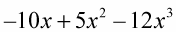##### Linear Algebra For DummiesWhen you use negative signs and multiple variables in algebraic equations, the problems can look scary. Don’t worry, though. All you need to do is distribute the negative sign through, changing the signs to their opposites. And remember that only the variables that are alike have exponential changes because the bases are the same.

The following problem is cluttered with negative signs.

Example: –4xyzw(4 – xyzw)

1. Multiply each term by –4xyzw.

–4xyzw(4) – 4xyzw(–x) – 4xyzw(–y) – 4xyzw(–z) – 4xyzw(–w)

2. Complete the multiplication in each term to complete the distribution.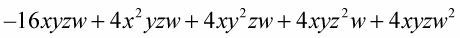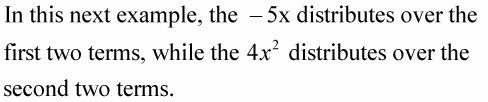Example: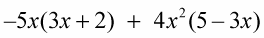1. Distribute the two different factors.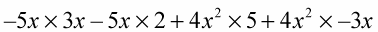2. Multiply the individual terms.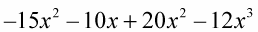3. The two terms with x2 combine into one term.